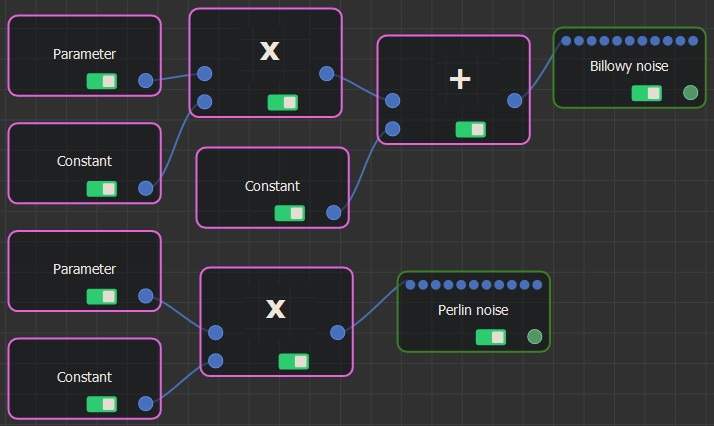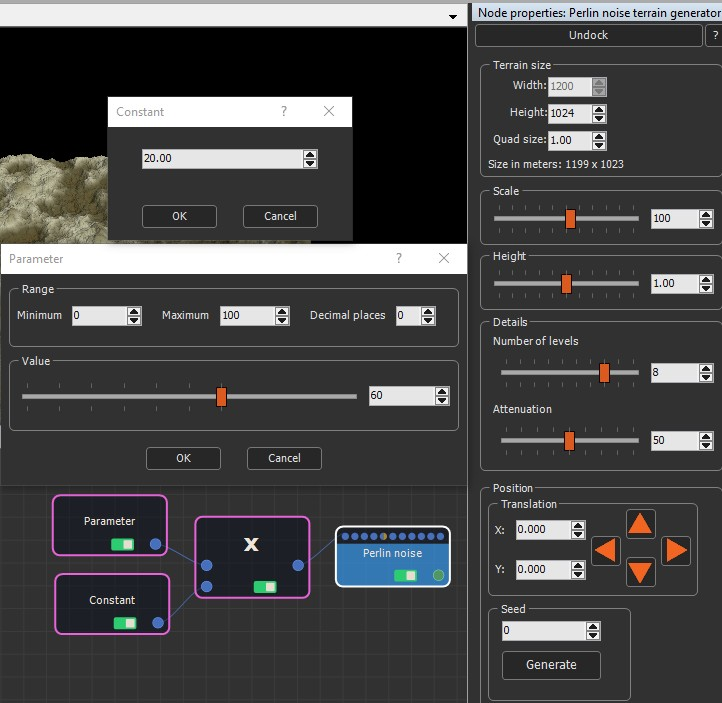### Adding a Multiply node

The Multiply node multiplies two input numbers to generate an output number. It is an alternative to the Formula node, but allows you to code a formula as a graph rather than a formula.

It has two input connectors and one output connector and multiplies the first input value with the second input value.

To add a Multiply node, select Edit in the main menu or right-click in the Graph Editor and select Create Node  > Calculation  > Multiply.The Multiply node has no parameters.

### Linking a Multiply node

The Multiply node input connectors link to two input Calculation nodes and the output connector to a Calculation node or an optional connector.### Example

In the example below, the Parameter value and a Constant value are multiplied to drive the terrain size of the Perlin noise. Note that the Terrain size value in the Perlin noise parameters is grayed out because it is replaced by the multiplied values.Copyright © 2021 · All Rights Reserved · Wysilab